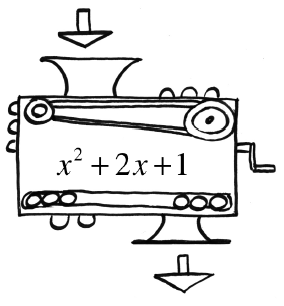### Home > INT3 > Chapter Ch1 > Lesson 1.1.3 > Problem1-45

1-45.

If $1$ is the output for Carmichael’s function machine shown at right, how can you determine what number is dropped in? Calculate the number(s) that could have been dropped in.

Since $1$ is the output, set the expression inside of the function machine equal to $1$.

$x^{2} + 2x + 1 = 1$

$x^{2} + 2x = 0$

$x\left(x + 2\right) = 0$

Use the Zero Product Property: $x = 0, x = −2$